# How to Calculate and Solve for Average Inflow Rate | Hydrology II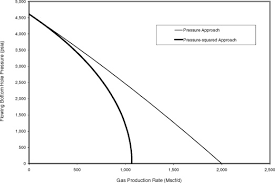The image above represents average inflow rate.

To compute for average inflow rate, three essential parameters are needed and these parameters are Average outflow rate (O), Change in storage during routing time (Δs) and Routing Time (Δt).

The formula for calculating average inflow rate:

I = O + Δs / Δt

Where:

I = Average Inflow Rate
O = Average Outflow Rate
Δs = Change in Storage during Routing Time
Δt = Routing Time

Let’s solve an example;
Find the Average Inflow Rate when the average outflow rate is 10, the change in storage during routing time is 5 and the routing time is 12.

This implies that;

O = Average Outflow Rate = 10
Δs = Change in Storage during Routing Time = 5
Δt = Routing Time = 12

I = O + Δs / Δt
I = 10 + 5 / 12
I = 10 + 0.416
I = 10.416

Therefore, the average inflow rate is 10.416.

Calculating the Average Outflow Rate when the Average Inflow Rate, the Change in Storage during Routing Time and the Routing Time is Given.

O = I – Δs / Δt

Where;

O = Average Outflow Rate
I = Average Inflow Rate
Δs = Change in Storage during Routing Time
Δt = Routing Time

Let’s solve an example;
Find the Average Outflow Rate when the average inflow rate is 50, change in storage during routing time is 20 and the routing time is 10.

This implies that;

I = Average Inflow Rate = 50
Δs = Change in Storage during Routing Time =20
Δt = Routing Time = 10

O = I – Δs / Δt
O = 50 – 20 / 10
O = 50 – 2
O = 48

Therefore, the average outflow rate is 48.

Calculating the Change in Storage during Routing Time when the Average Inflow Rate, the Average Outflow Rate and the Routing Time is Given.

Δs = (I – O) Δt

Where;

Δs = Change in Storage during Routing Time
I = Average Inflow Rate
O = Average Outflow Rate
Δt = Routing Time

Let’s solve an example;
Find the change in storage during routing time when the average inflow rate is 25, the average outflow rate is 15 and the routing time is 30.

This implies that;

I = Average Inflow Rate = 25
O = Average Outflow Rate = 15
Δt = Routing Time = 30

Δs = (I – O) Δt
Δs = (25 – 15) 30
Δs = (10) 30
Δs = 300

Therefore, the change in storage during routing time is 300.

Calculating for Routing Time when the Average Inflow Rate, the Average Outflow Rate and the Change in Storage During Routing Time is Given.

Δt = Δs / (I – O)

Where;

Δt = Routing Time
I = Average Inflow Rate
O = Average Outflow Rate
Δs = Change in Storage during Routing Time

Let’s solve an example;
Find the routing time when the average inflow rate is 25, the average outflow rate is 15 and the change in storage during routing time is 30.

This implies that;

I = Average Inflow Rate = 25
O = Average Outflow Rate = 15
Δs = Change in Storage during Routing Time = 30

Δt = Δs / (I – O)
Δt = 30 / (25 – 15)
Δt = 30 / (10)
Δt = 3

Therefore, the routing time is 3.

Nickzom Calculator – The Calculator Encyclopedia is capable of calculating the average inflow rate.

To get the answer and workings of the average inflow rate using the Nickzom Calculator – The Calculator Encyclopedia. First, you need to obtain the app.

You can get this app via any of these means:

To get access to the professional version via web, you need to register and subscribe for NGN 2,000 per annum to have utter access to all functionalities.
You can also try the demo version via https://www.nickzom.org/calculator

Apple (Paid) – https://itunes.apple.com/us/app/nickzom-calculator/id1331162702?mt=8
Once, you have obtained the calculator encyclopedia app, proceed to the Calculator Map, then click on Agricultural under Engineering.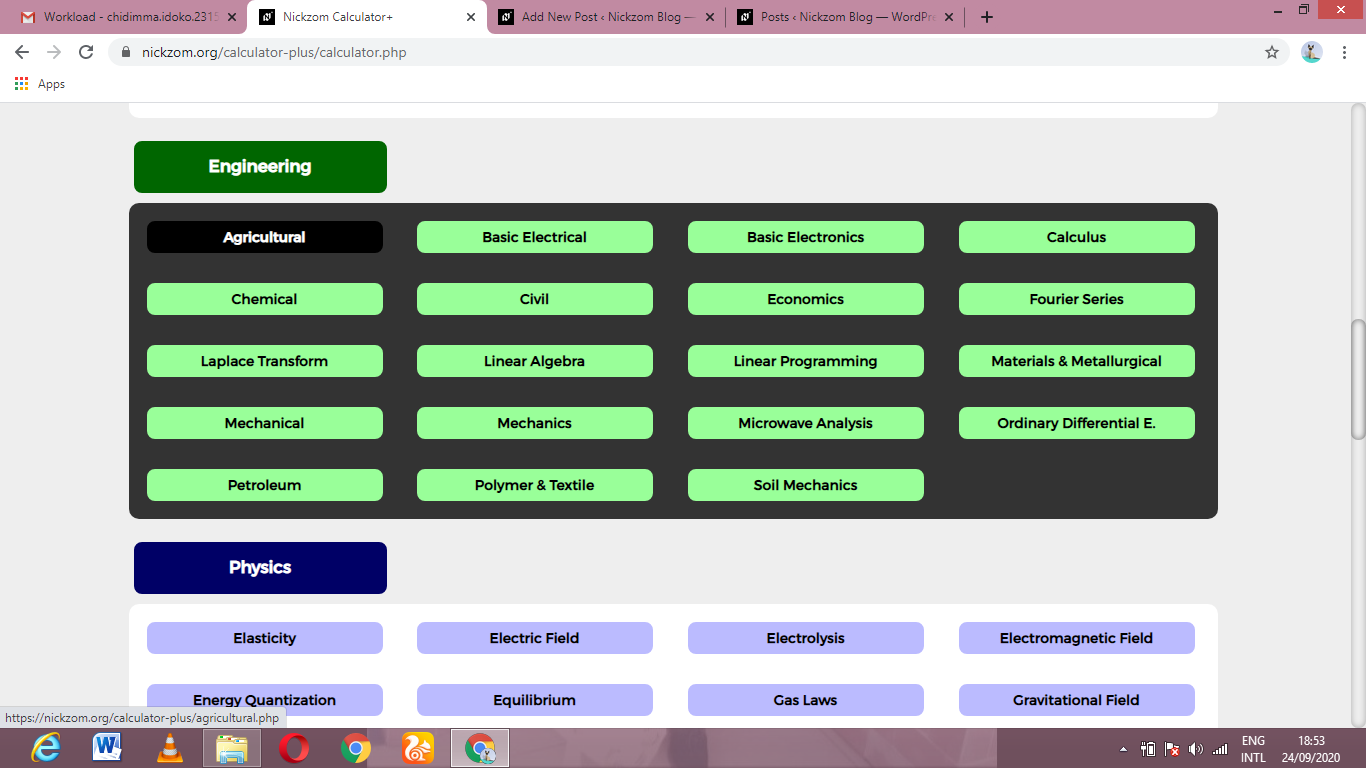Now, Click on Hydrology II under AgriculturalNow, Click on Average Inflow Rate under Hydrology II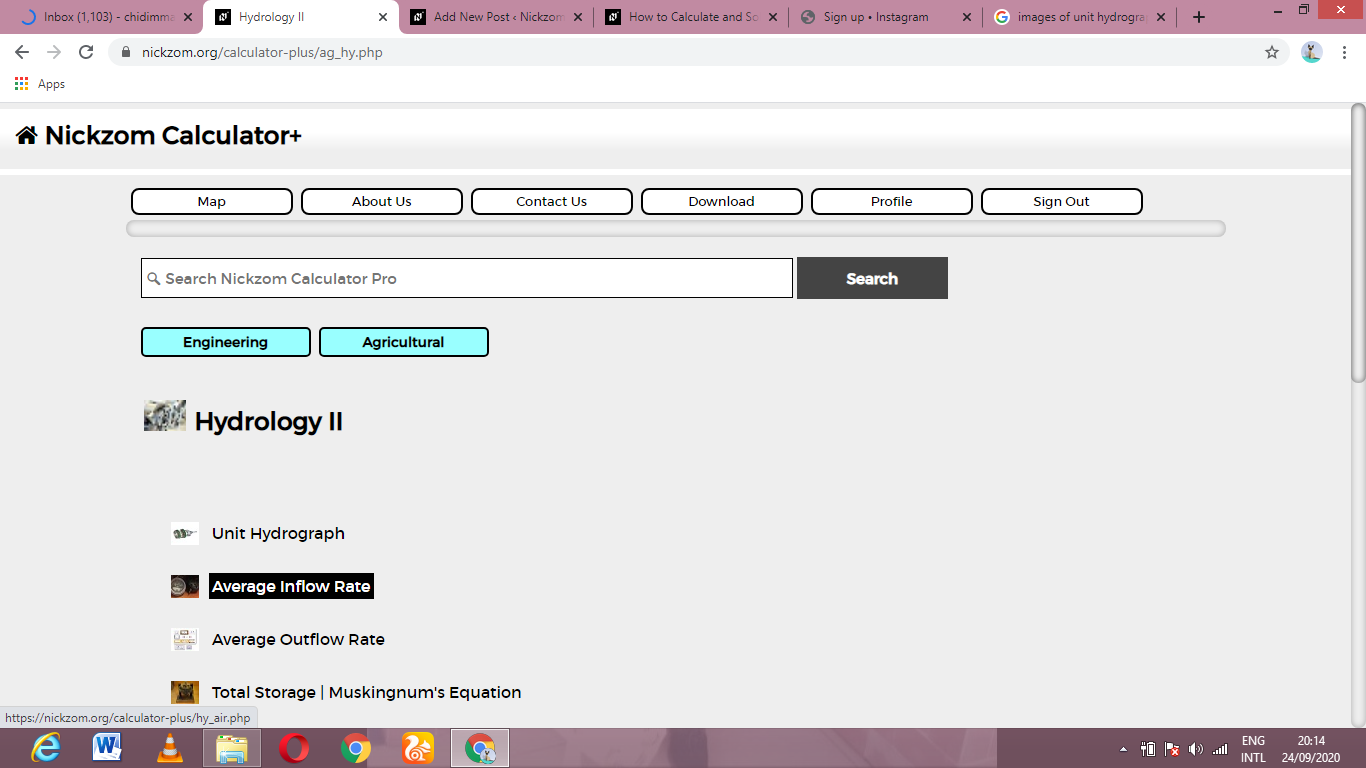The screenshot below displays the page or activity to enter your values, to get the answer for the average inflow rate according to the respective parameters which is the Average outflow rate (O), Change in storage during routing time (Δs) and Routing Time (Δt).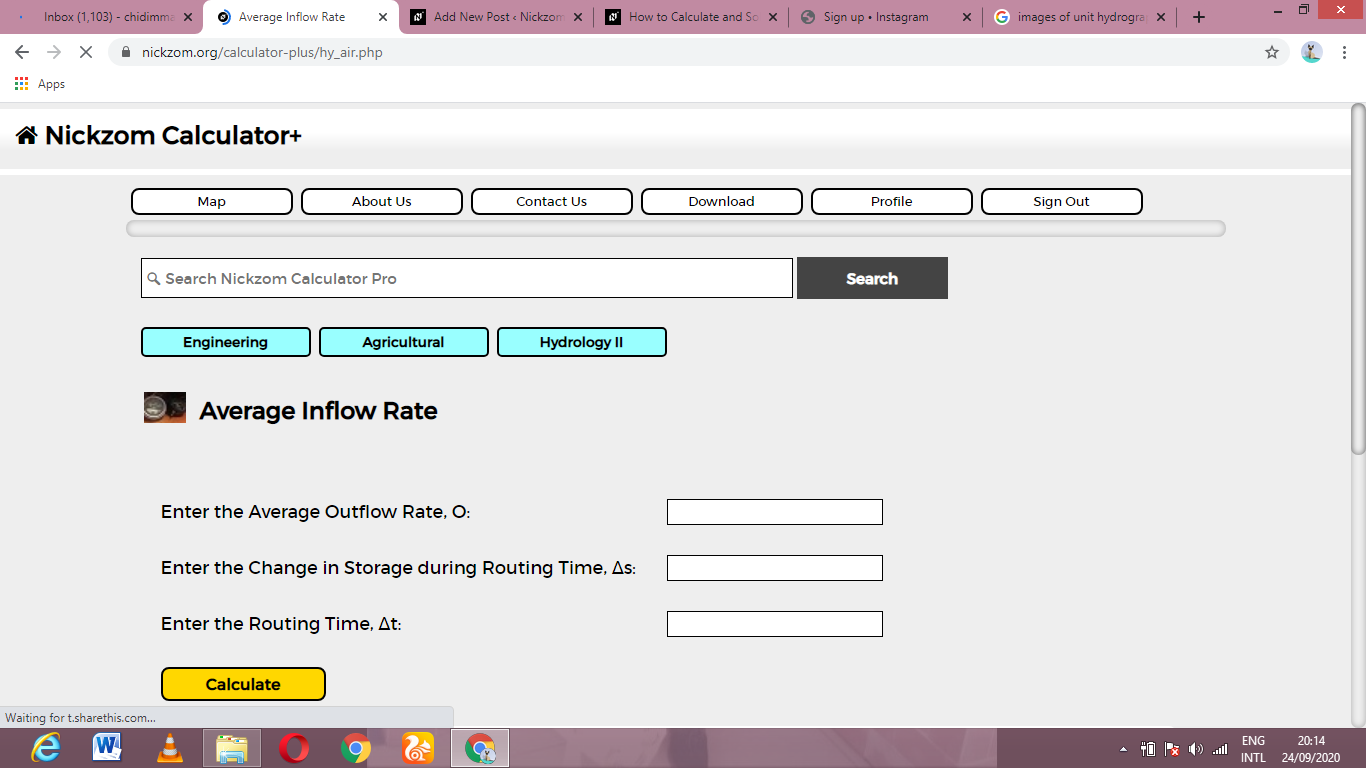Now, enter the values appropriately and accordingly for the parameters as required by the Average outflow rate (O) is 10, Change in storage during routing time (Δs) is 5 and Routing Time (Δt) is 12.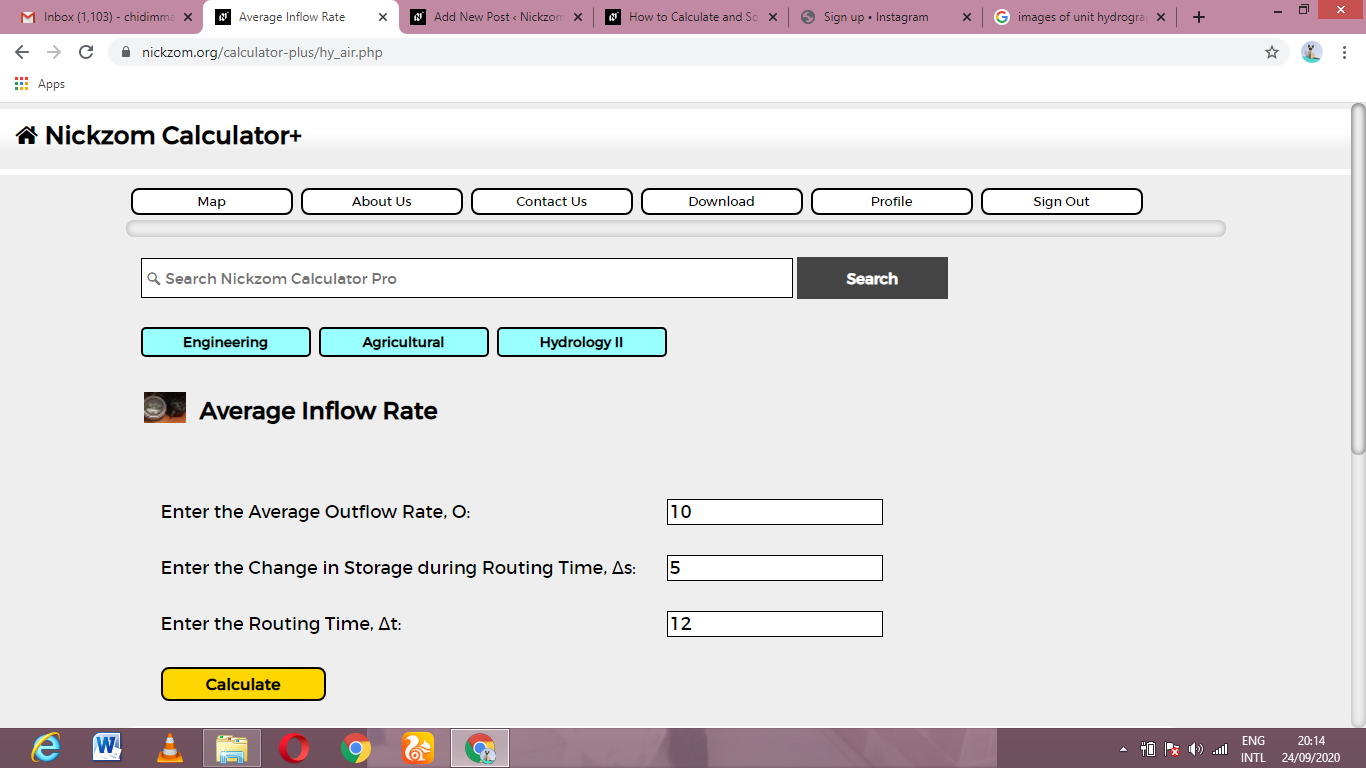Finally. Click on Calculate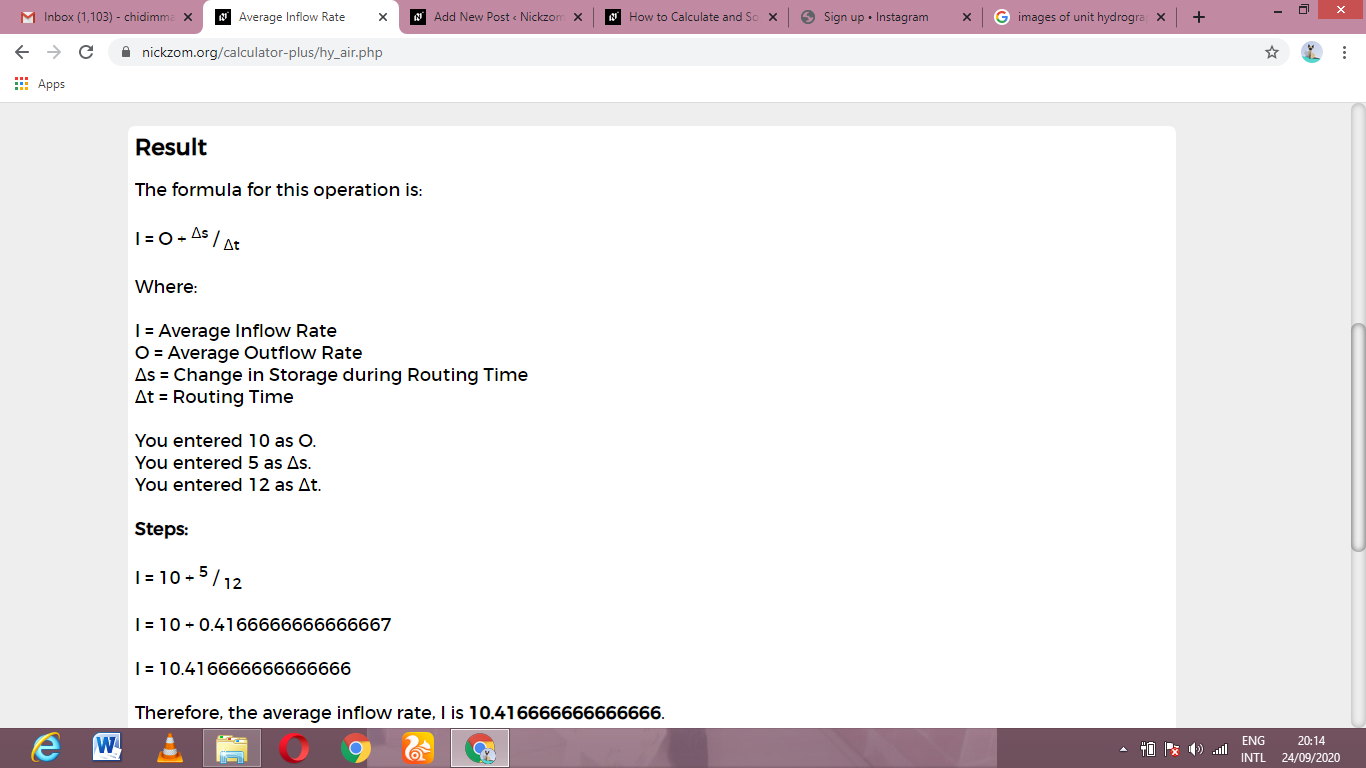As you can see from the screenshot above, Nickzom Calculator– The Calculator Encyclopedia solves for the average inflow rate and presents the formula, workings and steps too.